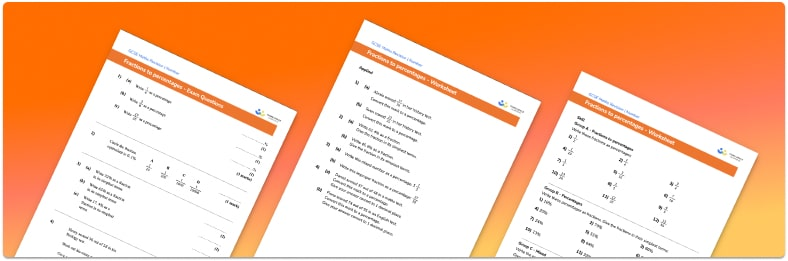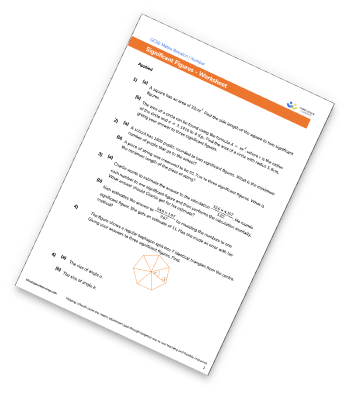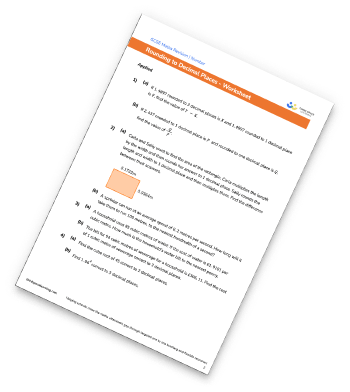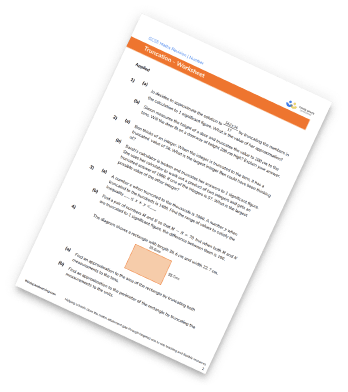# Converting Fractions To Percentages Worksheet• Section 1 of the converting fractions to percentages worksheet contains 36 skills-based converting fractions to percentages questions, in 3 groups to support differentiation
• Section 2 contains 4 applied converting fractions to percentages questions with a mix of word problems and deeper problem solving questions
• Section 3 contains 4 foundation and higher level GCSE exam style converting fractions to percentages questions
• Answer keys and a mark scheme for all converting fractions to percentages questions are provided
• Questions follow variation theory with plenty of opportunities for students to work independently at their own level
• All questions created by fully qualified expert secondary maths teachers
• Suitable for GCSE maths revision for AQA, OCR and Edexcel exam boards

• This field is for validation purposes and should be left unchanged.

You can unsubscribe at any time (each email we send will contain an easy way to unsubscribe). To find out more about how we use your data, see our privacy policy.

### Converting fractions to percentages at a glance

Percentages fractions and decimals are different formats for representing a part of a whole.

To convert a percentage to a fraction we can write the percentage as the numerator of the fraction and write the denominator as 100 (as percent means out of 100). We usually give fractions in their simplest form and so we need to simplify any fraction by cancelling common factors of the numerator and denominator.

To convert a percentage to a fraction we can use two different methods. One method is to find an equivalent fraction with a denominator of 100 which will give us the percentage as the numerator. Alternatively we could divide the numerator by the denominator, giving us the equivalent decimal, and then multiply by 100 to get the percentage.

Looking forward, students can then progress to additional number worksheets, for example aor aFor more teaching and learning support on Number our GCSE maths lessons provide step by step support for all GCSE maths concepts.

## Related worksheets

Significant Figures WorksheetRounding To The Nearest 10, 100, And 1000 WorksheetRounding Decimals WorksheetTruncation Worksheet## Do you have KS4 students who need more focused attention to succeed at GCSE?There will be students in your class who require individual attention to help them succeed in their maths GCSEs. In a class of 30, it’s not always easy to provide.

Help your students feel confident with exam-style questions and the strategies they’ll need to answer them correctly with our dedicated GCSE maths revision programme.

Lessons are selected to provide support where each student needs it most, and specially-trained GCSE maths tutors adapt the pitch and pace of each lesson. This ensures a personalised revision programme that raises grades and boosts confidence.

Find out more